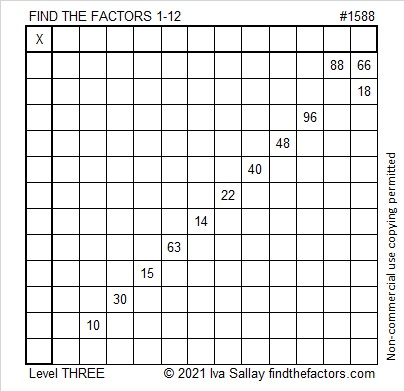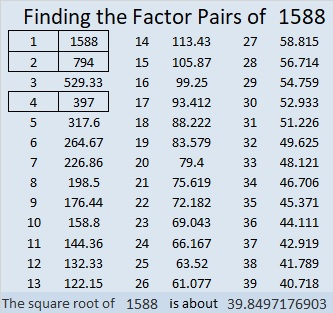# 1588 Cupid’s Arrow

### Today’s Puzzle:

Keeping with our Valentine’s theme, today’s level 3 puzzle looks like Cupid’s Arrow. Start with the clues at the top of the arrow, write in their factors, and work your way down the puzzle, cell by cell, writing in factors as you go. Before long, you will be smitten with this puzzle!### Factors of 1588:

• 1588 is a composite number.
• Prime factorization: 1588 = 2 × 2 × 397, which can be written 1588 = 2² × 397.
• 1588 has at least one exponent greater than 1 in its prime factorization so √1588 can be simplified. Taking the factor pair from the factor pair table below with the largest square number factor, we get √1588 = (√4)(√397) = 2√397.
• The exponents in the prime factorization are 2 and 1. Adding one to each exponent and multiplying we get (2 + 1)(1 + 1) = 3 × 2 = 6. Therefore 1588 has exactly 6 factors.
• The factors of 1588 are outlined with their factor pair partners in the graphic below.### More about the number 1588:

1588 is the difference of two squares:
398² – 396² = 1588.

1588 is also the sum of two squares:
38² + 12² = 1588.

1588 is the hypotenuse of a Pythagorean triple:
912-1300-1588, calculated from 2(38)(12), 38² – 12², 38² + 12².
It is also 4 times (228-325-397).